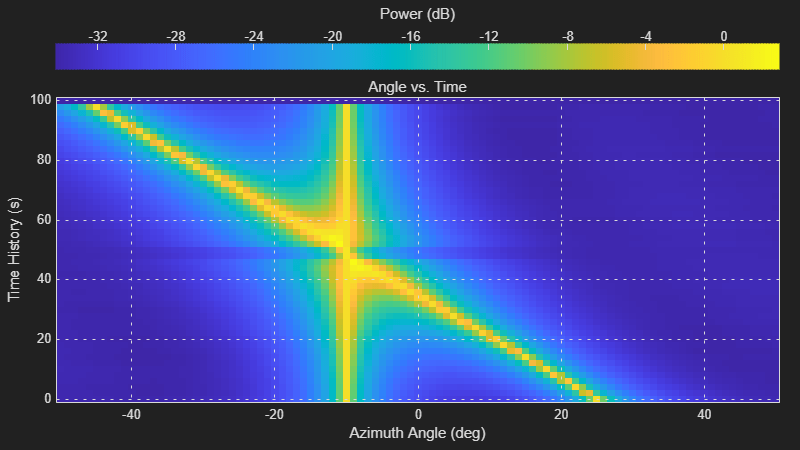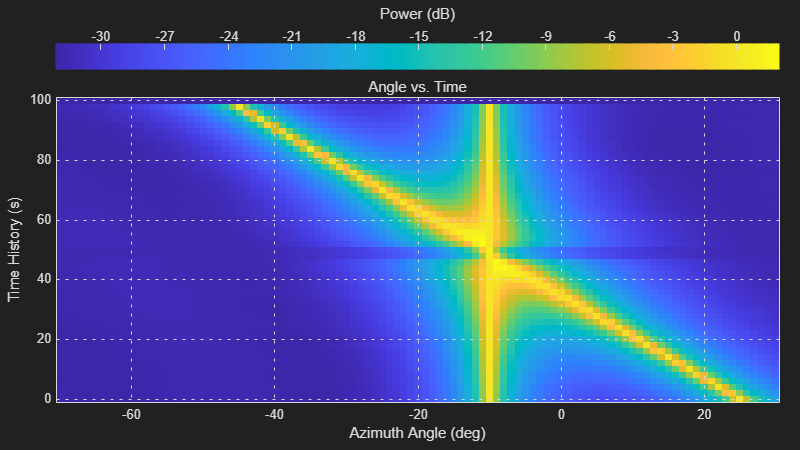# phased.ATIScope

View angle-time array response

Since R2022b

## Description

The `phased.ATIScope` System object™ creates an angle-time intensity (ATI) scope for displaying array response intensities as a function of time and angle of arrival. You can input two types of data – in-phase/quadrature (I/Q) data or intensity data. In either case, the scope displays signal magnitude or signal power.

• I/Q data – The data consists of fast-time I/Q samples of pulses and sweeps from multiple sensors. The scope creates intensity data and displays the array response map. To use I/Q data, set the `IQDataInput` property to `true`. In this mode, you can set the properties shown in Properties Applicable for Processed I/Q Data.

• Intensity data – The data consists of angle-time array intensity data. The scope displays the angle-time intensity response map. For example, you can obtain time-angle intensity data from the output of a beamformer. To use intensity data, set the `IQDataInput` property to `false`. In this mode, you can set the properties shown in Properties Applicable for Viewing Response Data.

To display angle-time response data:

1. Create the `phased.ATIScope` object and set its properties.

2. Call the object with arguments, as if it were a function.

## Creation

### Syntax

``scope = phased.ATIScope()``
``scope = phased.ATIScope(Name=Value)``

### Description

````scope = phased.ATIScope()` creates an ATI System object for displaying the angle-time intensity response of an array.```

example

````scope = phased.ATIScope(Name=Value)` creates an ATI `scope` System object with each specified property set to the specified value. See Properties for a list of properties. You can specify additional name-value pair arguments in any order as (`Name1`=`Value1`, … ,`NameN`=`ValueN`). For example,scope = phased.ATIScope(IQDataInput=true,IntensityUnits="power", ... SensorArray=phased.URA(Size=[4,3]),OperatingFrequency=1e6)creates an ATI scope System object that process I/Q data from a 4-by-3 uniform rectangular array. The intensity is displayed in power units. The operating frequency is 1 MHz.```

## Properties

expand all

Unless otherwise indicated, properties are nontunable, which means you cannot change their values after calling the object. Objects lock when you call them, and the `release` function unlocks them.

If a property is tunable, you can change its value at any time.

Scope window name, specified as a character vector or string. The name appears in the title bar of the window.

Example: `'Multi-target Angle-Time Intensity Scope'`

Tunable: Yes

Data Types: `char` | `string`

Location and size of the intensity scope window, specified as a 1-by-4 vector having the form `[left bottom width height]`.

• `left` and `bottom` specify the location of the bottom-left corner of the window.

• `width` and `height` specify the width and height of the window.

Units are in pixels.

The default value of this property depends on the resolution of your display. By default, the window is positioned in the center of the screen with a `width` of 800 pixels and a `height` of 450 pixels.

Example: `[100 100 500 400]`

Tunable: Yes

Data Types: `double`

Input data type, specified as `false` or `true`. When `true`, the input consists of complex I/Q sample data and further processing is required to transform to the angle-time domain. When `false`, the input data is real response data that has already been transformed to the angle-time domain.

Data Types: `logical`

Angle-axis label, specified as a character vector or a string.

Example: `'Angles (rad)'`

Tunable: Yes

Data Types: `char` | `string`

Angle separation between samples, specified as a positive scalar. This property defines the angle difference between columns of the input matrix. Units are in degrees.

#### Dependencies

To enable this property, set the `IQDataInput` property to `false`.

Data Types: `double`

Angle offset, specified as a scalar. This property defines the angle value of the first column of the input matrix. Units are in degrees.

#### Dependencies

To enable this property, set the `IQDataInput` property to `false`.

Data Types: `double`

Time interval between samples, specified as a positive scalar. This property defines the time interval between the rows of the scope. Units are in seconds.

Data Types: `double`

Time span of the intensity display, specified as a positive scalar. Units are in seconds.

Data Types: `double`

Response intensity units, specified as `'db'`, `'magnitude'`, or `'power'`.

Data Types: `char` | `string`

Display title, specified as a character vector or string.

Tunable: Yes

Data Types: `char` | `string`

Sensor array, specified as a Phased Array System Toolbox array System object. See `phased.ULA` for the default values of a uniform linear array.

Example: `phased.URA`

#### Dependencies

To enable this property, set the `IQDataInput` to `true`.

Direction of arrival estimation method, specified as `'beamscan'`, `'mvdr'`, or `'music'`.

Example: `'mvdr'`

#### Dependencies

To enable this property, set the `IQDataInput` property to `true`.

Data Types: `char` | `string`

Signal propagation speed, specified as a positive scalar. The default value of this property is the speed of light. See `physconst`. Units are in meters/second.

Example: `3e8`

#### Dependencies

To enable this property, set the `IQDataInput` property to `true`.

Data Types: `double`

Operating frequency of the system, specified as a positive scalar. Units are in Hz.

Example: 2e9

#### Dependencies

To enable this property, set the `IQDataInput` property to `true`.

Data Types: `double`

The number of bits used to quantize the phase shift of the applied beamformer, specified as a non-negative integer. A value of zero indicates that no quantization is performed.

Example: `5`

#### Dependencies

To enable this property, set the `IQDataInput` property to `true`.

Data Types: `double`

Set this property to `true` to allow forward-backward averaging to estimate the covariance matrix for sensor arrays having a conjugate-symmetric array manifold.

#### Dependencies

To enable this property, set the `IQDataInput` property to `true`.

Data Types: `logical`

Azimuth scan angles, specified as a scalar or real-valued row vector. The angles must lie between –180° and 180°, inclusive. Specify the angles in increasing order. At least one of the sets of angles defined in the `AzimuthScanAngles` and `ElevationScanAngles` properties must be a scalar. Units are in degrees.

Example: `-20:20`

#### Dependencies

To enable this property, set the `IQDataInput` property to `true`.

Data Types: `double`

Elevation scan angles, specified as a scalar or real-valued row vector. The angles must lie between –90° and 90°, inclusive. Specify the angles in increasing order. At least one of the sets of angles defined in the `AzimuthScanAngles` and `ElevationScanAngles` properties must be a scalar. Units are in degrees.

#### Dependencies

To enable this property, set the `IQDataInput` property to `true`.

Data Types: `double`

## Usage

### Syntax

``scope(X)``

### Description

example

````scope(X)` displays an ATI scope of array response intensities derived from the data `X`. You can display magnitude or power values.```

### Input Arguments

expand all

• When the `IQDataInput` property is `false`, `X` represents intensity data, specified as an M-by-N real-valued matrix. Each column of the matrix represents an intensity vector from successive times. Each matrix row M is the number of intensity values in an angle intensity vector and N is the number of intensity vectors. M should be greater than 1 and N should be equal to or greater than 1. The time between the intensity vectors is specified in the `TimeResolution` property.

• When the `IQDataInput` property is `true`, `X` represents I/Q data, specified as an M-by-N complex-valued matrix. Each row contains the data samples for all sensors at a single time. Each column contains the I/Q data for one sensor for all times.

Data Types: `double`
Complex Number Support: Yes

## Object Functions

To use an object function, specify the System object as the first input argument. For example, to release system resources of a System object named `obj`, use this syntax:

`release(obj)`

expand all

 `hide` Turn off visibility of scope `isVisible` Visibility of scopes `show` Turn on visibility of scopes
 `step` Run System object algorithm `release` Release resources and allow changes to System object property values and input characteristics `reset` Reset internal states of System object

## Examples

collapse all

Display the directions-of-arrival (DOA) of two signals arriving at a 10-by-5 uniform rectangular array (URA).

First, create a `phased.URA` array System object™ containing `phased.IsotropicAntennaElement` System objects spaced 0.45 wavelengths apart.

```fc = 200e6; lambda = physconst('LightSpeed')/fc; d = 0.45*lambda; element = phased.IsotropicAntennaElement( ... FrequencyRange=[100e6,300e6]); array = phased.URA(Element=element,Size=[10,5], ... ElementSpacing=[d,d]);```

Then, create an ATIScope to process I/Q data.

```scope = phased.ATIScope(IQDataInput=true, ... SensorArray=array, ... DOAMethod="mvdr", ... OperatingFrequency=fc, ... AzimuthScanAngles=-50:50, ... ElevationScanAngles=0, ... AngleLabel="Azimuth Angle (deg)", ... TimeSpan=100, ... TimeResolution=2);```

Generate baseband signals at 300 and 400 Hz. The sampling rate of the baseband signals is 8 kHz.

```fs = 8000; t = (0:1/fs:1).'; x1 = exp(-1i*2*pi*t*300); x2 = exp(-1i*2*pi*t*400);```

Create two paths in azimuth-elevation space. The first path is a straight line starting at -45 degrees in azimuth and stopping at 25 degrees with 50 samples. The second path has constant azimuth of 30 degrees.

```path1 = [linspace(-45,25,50); 0.0*ones(1,50)]; path2 = [-10*ones(1,50);zeros(1,50)]; xtot = [x1,x2]; for k = 1:size(path2,2) azel1 = [path1(:,k),path2(:,k)]; x = collectPlaneWave(array,xtot,azel1,fc); noise = 0.1*(randn(size(x)) + 1i*randn(size(x))); scope(x + noise); end```Display the directions-of-arrival (DOA) of two signals arriving at a 10-by-5 uniform rectangular array (URA).

First, create a `phased.URA` array System object™ composed of `phased.IsotropicAntennaElement` System objects.

```fc = 200e6; lambda = physconst('LightSpeed')/fc; d = 0.45*lambda; element = phased.IsotropicAntennaElement(FrequencyRange=[100e6,300e6]); array = phased.URA(Element=element,Size=[5,5],ElementSpacing=[d,d]);```

Then, create a `phased.ATIScope` System object.

```scope = phased.ATIScope(IQDataInput=false, ... AngleLabel="Azimuth Angle (deg)", ... TimeSpan=100,TimeResolution=2, ... AngleOffset=-70);```

Generate baseband signals at 300 and 400 Hz. The sampling rate of the baseband signal is 8 kHz.

```fs = 8000; t = (0:1/fs:1).'; x1 = exp(-1i*2*pi*t*300); x2 = exp(-1i*2*pi*t*400);```

Create an MVDR beamformer System object. Specify the beamformer pointing angles.

```azAngle = -50:50; elAngle = zeros(size(azAngle)); bfAngle = [azAngle;elAngle]; beamformer = phased.MVDRBeamformer(SensorArray=array, ... PropagationSpeed=physconst('LightSpeed'), ... OperatingFrequency=fc,Direction=bfAngle, ... WeightsOutputPort=false);```

Create two of objects in azimuth-elevation space. The first path is a straight line starting at $-{45}^{\circ }$ in azimuth and ending at ${25}^{\circ }$. The second path is at a constant azimuth of ${10}^{\circ }$ degrees. Both paths have 50 samples.

```path1 = [linspace(-45,25,50);zeros(1,50)]; path2 = [-10*ones(1,50);zeros(1,50)]; xtot = [x1,x2]; ymn = zeros(size(bfAngle,2)); for k = 1:size(path2,2) azel1 = [path1(:,k),path2(:,k)]; x = collectPlaneWave(array,xtot,azel1,fc); noise = 0.1*(randn(size(x)) + 1i*randn(size(x))); y = beamformer(conj(x) + noise); ymn(k,:) = mean(abs(y),1); scope(ymn) end scope(ymn)```